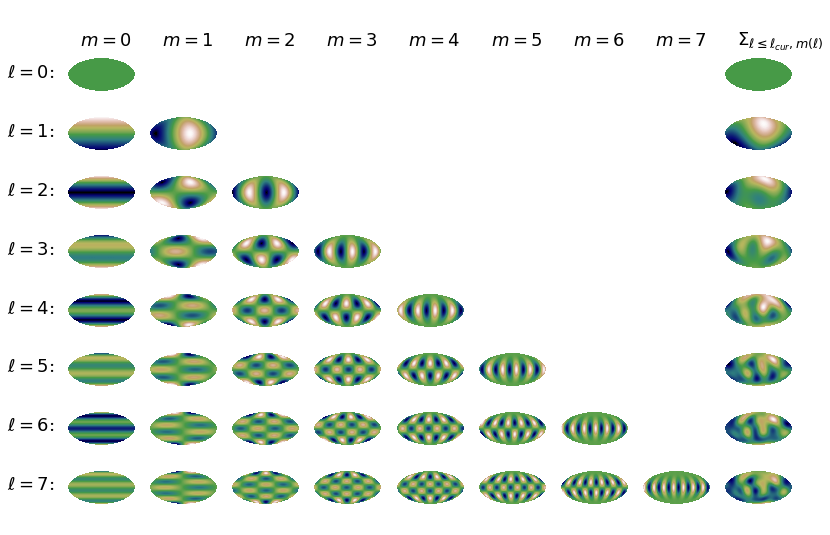These pages have been developed for the ‘Girls' Day 2022’ – an outreach event for pupils – by Fabio D'Ambrosio, Iris Feldt, Eileen Giesel, Lavinia Heisenberg, David Maibach, Sara Marcos Plaza, Nadine Nussbaumer, Alexander Oestreicher, Adrian Schirra, Sofía Vidal, Ricardo Waibel, and Stefan Zentarra, based on the Girls' Day 2021 pages. In this context, also this video was created, which explains the work in our group.

# The cosmic microwave background and spherical harmonics

page    ⟨  1    2  ⟩

### 1) Map of the Earth

Here you can see on the right-hand side a map of the Earth. The colours represent the altitude above sea level in metres m>

.

Some of the regions have negative values – what does this mean?

Are you surprised by the figure?

What would happen, if one crossed the right edge of the map?### 2) Reconstruct the altitudes of the Earth surface

On the right-hand side you can see once again a map of the Earth – at least if you drag the slider all the way to the right.

How does the image of the Earth change, when you change the value of ℓ to smaller or larger values?

What is the role of the ℓ?

You can see a so-called ‘power spectrum’ on the left-hand side. Such power spectra are also used in cosmology. The white part of the plot marks the range of ℓ that has been used for reconstructing the map of the Earth on the right-hand side. All scales higher than the ℓ you have set are ignored and thus marked by the grey region. The more information we use, that means the more scales we take into account for reconstructing the map of the Earth’s altitudes, the more precise we can reproduce the original image.

ℓ :### 3) How does the reconstruction work? What is the role of ℓ?

In the following figure, you can see the ‘spherical harmonics’. They are used as basis functions for the reconstruction of the original image. Why do we actually need functions that are two-dimensional? Do you understand why they are called spherical harmonics? Why the ‘spherical’ and why the ‘harmonic’?

As you can see in the triangular figure below, there exist one or several different basis functions for each ℓ (see the rows). How many basis functions are there for ℓ = 1? How do the patterns of the basis functions change when ℓ is increased?

In the last column on the right-hand side, you can see what happens when we weight all basis functions up to this ℓ and add them toghether. By the way, with weighting we mean that we multiply the basis functions by a factor that is the heigher the more important this basis function is.

You see again, the more basis functions we take into account, the more precise the reconstruction can get.

… but where do we know the weighting factors of the basis functions from? (see part 3b and 3c)Here you can select yourself which basis functions you would like to add and how this changes the image. The functions are already multiplied by the correct weighting factor.### 3b) Where do we know the weighting factors from?

For a one-dimensional signal, sine functions are the analogy of spherical harmonics. In the figure you can see such a sine function with two variable parameters A and B. Change those two values, what do they mean?

A:

B:

By a superposition of different sine functions we can create arbitrary signals, like the red one below. The blue functions in this game are the basis functions. They can be multiplied by the amplitudes (or prefactors) A, B, C and added. The sum results in the black curve. Can you adjust the prefactors such that the black curve reproduces the ‘measured’ red signal?

A:

B:

C:

### 3c) Prefactors in 2D?

Going back to our earth, we have to look at spherical harmonics instead of sine curves. Below on the left you can see the partial sum of spherical harmonics, already with the calculated coefficients. This leaves three ingredients for the earth: the coarse structure (top), the medium sized structures (middle), and the fine strucure (bottom).

Can you choose the prefactors of these three ingredients in such a way, that the image of their sum on the right is the same as the one of our earth?

Coarse:   Medium:   Fine:

Canvas does not work in your browser. Change! Canvas does not work in your browser. Change!
Seite    ⟨  1    2  ⟩
Verantwortlich: Matthias Bartelmann